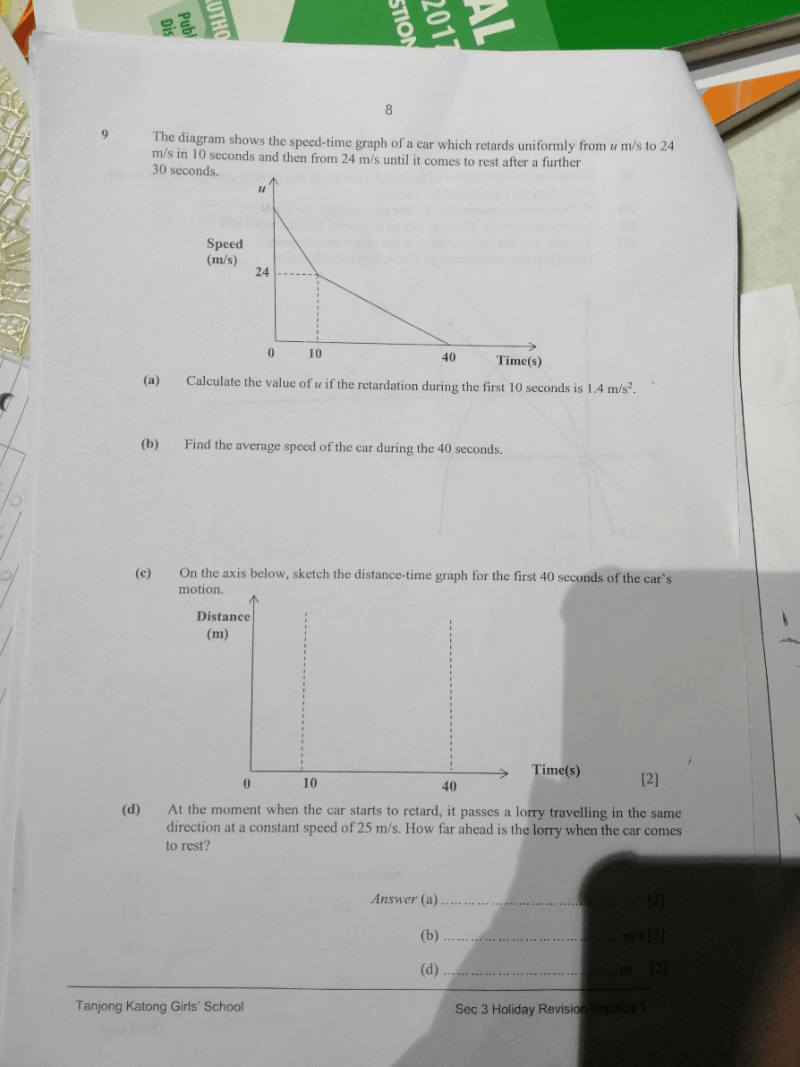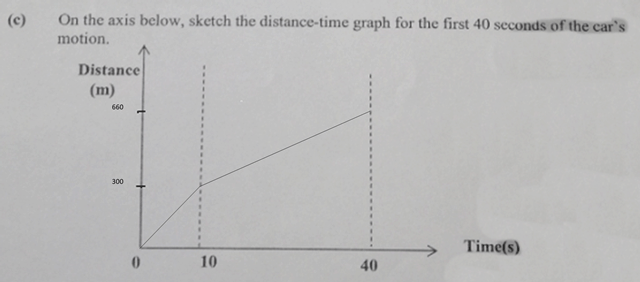# Question1 Answer

# Answera) Retardation of 1.4 m/s2 during the first 10 seconds means that the car travels 1.4 m/s slower every second. So for 10 seconds, his speed would have decreased a total of (1.4 x 10) m/s.

u (initial speed) → 24 + (1.4 x 10)
= 24 + 14
= 36 m/s

b) Average Speed = Total Distance / Total Time

The Total Distance is the area under the speed/time graph.

Distance for first 10 sec = Area of trapezium on the left
= 1/2 x (24 + 36) x 10
= 1/2 x 60 x 10
= 300 m

Distance for next 30 sec = Area of bottom right triangle
= 1/2 x 30 x 24
= 360 m

Total distance travelled → 300 + 360
= 660 m

Average Speed = 660 m / 40 s
= 16.5 m/s

c) See attached picture

d) Lorry travelled at constant speed, so for the entire 40 sec duration, it would have travelled:
25 m/s x 40 s = 1,000 m

We know that the car travelled a total of 660 m during the 40 sec, so the lorry would be (1,000 – 660) m ahead.

1000 – 660 = 340 m

0 Replies 0 Likes